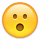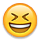# Half of my 6* roster is science. What are these odds?What are the odds of this even happening.
«1

• edited November 2020
1/162.

I think if you’re specifically talking about the hulk pull.
•What are the odds of this even happening.

Can u show ur whole roster if u don't mind ☺ just interested
• Lmao said:

1/162.

I think if you’re specifically talking about the hulk pull.

I don't think it's 1/162. Shouldn't it be 0.019897
169 champs available as 6*s. 7 are not in the basic yet.
• Lmao said:

1/162.

I think if you’re specifically talking about the hulk pull.

I don't think it's 1/162. Shouldn't it be 0.019897
169 champs available as 6*s. 7 are not in the basic yet.
But he's asking what are the odds of pulling 5 science champs right? not just the hulk
• edited November 2020

The odds are one thing. The amount this occurs is another. It's very common for Rosters to lean to one side, or not lean to another. Eventually, they fill in.

What if they never fill in......... 😱
• Out of my 9 6 stars 5 are mutants and I managed to dupe one of the useless mutants so I consider that even worse.
• i have 0 6 stars but i do have 10 5 stars and 5 of them of science, 3 mystic 1 skill and 1 tech
•4/7
I win

your six star roster is crazy good. joe fixit and cyclops• Of my 62 six stars, only 7 of them are science. 12 tech, 11 mutant, 11 skill, 10 cosmic, 11 mystic.
The more you open, the more it starts to even out.
• Push said:

Assuming independence of event:
P(4 of 7 science) =
= P(sci)^4 * P(!sci)^3
= P(#of science in crystal / # in crystal)^4 *
P(1 - (#Sci / #crystal))^3

Why would you use this equation instead of a binomial distribution equation? I'm kinda confused
• Lmao said:

Push said:

Assuming independence of event:
P(4 of 7 science) =
= P(sci)^4 * P(!sci)^3
= P(#of science in crystal / # in crystal)^4 *
P(1 - (#Sci / #crystal))^3

Why would you use this equation instead of a binomial distribution equation? I'm kinda confused
Poisson distribution > binomial distribution
• edited November 2020
Kill_Grey said:

Lmao said:

Push said:

Assuming independence of event:
P(4 of 7 science) =
= P(sci)^4 * P(!sci)^3
= P(#of science in crystal / # in crystal)^4 *
P(1 - (#Sci / #crystal))^3

Why would you use this equation instead of a binomial distribution equation? I'm kinda confused
Poisson distribution > binomial distribution
This isn’t a poisson distribution tho
• Lmao said:

Kill_Grey said:

Lmao said:

Push said:

Assuming independence of event:
P(4 of 7 science) =
= P(sci)^4 * P(!sci)^3
= P(#of science in crystal / # in crystal)^4 *
P(1 - (#Sci / #crystal))^3

Why would you use this equation instead of a binomial distribution equation? I'm kinda confused
Poisson distribution > binomial distribution
This isn’t a poisson distribution tho
Or whatever it's called, lol.
• This happened with me when I was first pulling 5*s...the more you pull, the more it should balance out among the classes.
• edited November 2020
I have 10 and 5 are mutants. I feel your pain. Not a single science actually. 2 skill, one cosmic and one mystic and one tech.
• If you wanna talk about pain hear me out. I have around 40 6* and only one cosmic which is Gamora and guess what?

I have 2 cosmic t5CCs for more than 6 months now.One from first abyss run,the other from map7 etc.
• From the title I thought this was a joke post and you had 2 6* champions
• 12 out of 22 of my 6*'s are skill...
• My roster was like this at first, heavy on science and cosmic six stars, lacking any tech. Now it has mostly evened out, although science and cosmic are still ahead of the other classes.

All of these probability discussions are meaningless in terms of what you can expect to happen to you, BTW.
• I had the same issue and pulled 5 techs out of my first 9-10 6*s

Nebula
green goblin
Yondu
Vulture
Nebula
• You’re right. Essentially, a binomial distribution expands into this one if the the probabilities are the same and if I took the combinations into account.

I was thinking about how long it takes to get a 6* and how the crystal values changed.

A binomial with current odds is probably a decent estimate though.
Lmao said:

Push said:

Assuming independence of event:
P(4 of 7 science) =
= P(sci)^4 * P(!sci)^3
= P(#of science in crystal / # in crystal)^4 *
P(1 - (#Sci / #crystal))^3

Why would you use this equation instead of a binomial distribution equation? I'm kinda confused
• edited November 2020
Only one mutant and no science from my 16. Sitting on 50k shards and a rank 1 to 2 science gem and so reluctant to open them for fear of deep disappointment.. 4 cosmic and 4 tech?!If I had that roaster I would not care at all